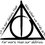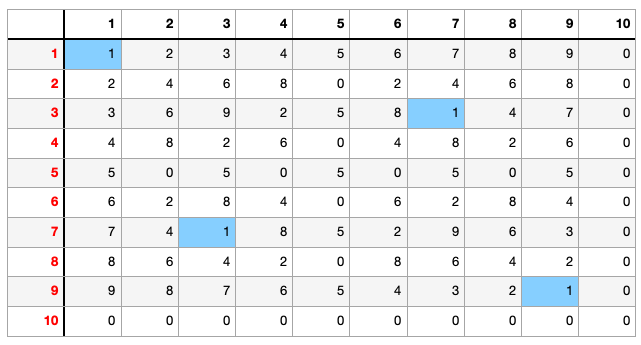# The poker card Qs

From a stack of poker cards, the cards A, 2, 3, 4, 5, 6, 7, 8, 9, 10 of spades and A, 2, 3, 4, 5, 6, 7, 8, 9, 10 of Hearts were selected.

Out of these twenty cards, how many ways can one select two cards-one from Hearts and one from spades-such that the product of the two numbers leaves a remainder of 1 when divided by 10?Note by Vicky Clearwater
1 year, 1 month ago

This discussion board is a place to discuss our Daily Challenges and the math and science related to those challenges. Explanations are more than just a solution — they should explain the steps and thinking strategies that you used to obtain the solution. Comments should further the discussion of math and science.

When posting on Brilliant:

• Use the emojis to react to an explanation, whether you're congratulating a job well done , or just really confused .
• Ask specific questions about the challenge or the steps in somebody's explanation. Well-posed questions can add a lot to the discussion, but posting "I don't understand!" doesn't help anyone.
• Try to contribute something new to the discussion, whether it is an extension, generalization or other idea related to the challenge.

MarkdownAppears as
*italics* or _italics_ italics
**bold** or __bold__ bold
- bulleted- list
• bulleted
• list
1. numbered2. list
1. numbered
2. list
Note: you must add a full line of space before and after lists for them to show up correctly
paragraph 1paragraph 2

paragraph 1

paragraph 2

[example link](https://brilliant.org)example link
> This is a quote
This is a quote
    # I indented these lines
# 4 spaces, and now they show
# up as a code block.

print "hello world"
# I indented these lines
# 4 spaces, and now they show
# up as a code block.

print "hello world"
MathAppears as
Remember to wrap math in $$ ... $$ or $ ... $ to ensure proper formatting.
2 \times 3 $2 \times 3$
2^{34} $2^{34}$
a_{i-1} $a_{i-1}$
\frac{2}{3} $\frac{2}{3}$
\sqrt{2} $\sqrt{2}$
\sum_{i=1}^3 $\sum_{i=1}^3$
\sin \theta $\sin \theta$
\boxed{123} $\boxed{123}$

Sort by:

A two-way table as such can be made. With each entry entered as product and then of $\mod {(10)}$. There are only 4 entries of 1- 1 year, 1 month ago

WOW thanks

- 1 year, 1 month ago

We must solve $xy \equiv 1$ mod 10.

For any $x$ that is not a zero divisor modulo 10 (i.e. not a multiple of 2 or 5), this equation has a unique solution modulo 10. For other $x$, there is no solution. Therefore there are $\phi(10) = 4$ solutions.

- 1 year, 1 month ago

Of course, this kind of quick argument only works if you know some abstract algebra.

If this is a high school assignment, you're best off reasoning through it in a different way (e.g. making a table like Mahdi did).

- 1 year, 1 month ago

How did you conclude that there are 4 solutions?

- 1 year, 1 month ago

Sorry, that's the part I forgot. I just edited my solution.

The Euler totient function $\phi(10) = \phi(2)\cdot \phi(5) = 1 \cdot 4 = 4$ gives the number of elements in the multiplicative group modulo 10, which is the number of non-zero divisors, as well as the number of invertible elements modulo 10.

- 1 year, 1 month ago

Can this be concluded for $2 \mod{(10)}$ as well.

- 1 year, 1 month ago

No. Since 2 is a zero divisor modulo 10 ($2\times 5 \equiv 0$), it is not an invertible element. You would reduce the equation to $x'y' \equiv 1 \ \ \ \text{mod}\ 5,$ which has $\phi(5) = 4$ solutions. Afterward, double either $x$ or $y$, and optionally add 5 to the other, resulting in 16 solutions. In the table below, the first column has the values of $(x',y')$: $\begin{array}{c|cccc} (1,1) & (2,1) & (1,2) & (2,6) & (6,2) \\ (2,3) & (4,3) & (2,6) & (4,8) & (7,6) \\ (3,2) & (6,2) & (3,4) & (6,7) & (8,4) \\ (4,4) & (8,4) & (4,8) & (8,9) & (9,8) \\ \end{array}$ There are some doubles here; the actual number of solutions is 12.

- 1 year, 1 month ago

Yeah, I now understand. This is a good way actually. Thanks!

- 1 year, 1 month ago

Note that the "doubles" are all of the form $(x,y) = (2u,2v)$, where $uv \equiv \frac12 \equiv 3\ \ \ \text{mod 5}.$ This equation has four solutions.

- 1 year, 1 month ago

But for the other non-zero divisors ($xy \equiv 1, 3, 7, 9$ mod 10) there are four solutions each.

- 1 year, 1 month ago